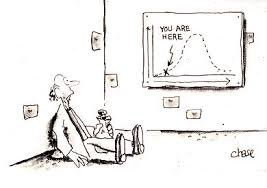# Normal Distribution Generator in excelsource: Fonlinecourses.science.psu.edu

In a study, sometimes we face a limited amount of data and data is not normally distribution. Whereas statistical data require much or at least 30 data to meet the parametric prerequisites. The question is whether allowing to use normal distribution generator with a limited numbers. The answer is allowed. Even if we only know the value of the mean (average) and standard deviation of the data, then we can perform simulations to 1000 data or more. For example, we know the value of the mean = 20 and the standard deviation is 5, and the sample from population that is normally distributed. To obtain normally distributed random numbers or a particular distribution. we can use Monte Carlo simulation using various software available.

The steps to simulate the limited data that follow a particular distribution pattern is as follows:

## 1.Define value starting point

To get the next random number requires starting point. However, the numbers starting point does not significantly affect the data simulation, because the starting point of this figure is just one figure among the thousands of numbers that will be obtained based on the simulation results.## 2. Determine the expected population distribution

Prior to the simulation data, we must determine the distribution assumption of population data that we expect. For example, we assume that the data will follow a normal distribution pattern.

We need to know the various types of distribution in accordance with scale of the data.

If the data is a numerical scale that allows below distribution: normal distribution, log-normal, exponential, and others.

Meanwhile, if the scale is categorical, so the distribution are: binomial distribution, uniform distribution, multinomial distribution, hiper geomertric distribution and so on.3. Determine the required assumptions for population distribution

Every distribution has certain statistical parameters. For example, if we assume a normal distribution, then at least we should know two parameters: mean and standard deviation. these two parameters will be used to generate other data.4. Running data based on the assumption

After determining the necessary assumptions, the next process is running data. We can process/running using iteration until 1000 and even up to more than 1000 times. If we do a running 1000 times then we will get 1000 random numbers that follow the pattern of distribution that we choose.5. Make reports

Once the data is complete for running, then the output can be clicked to display the output of any report requiredThe result is that we will get random numbers 1000 data that follow certain patterns of distribution, such as the normal distribution. Obviously the parameters mean (average) and standard deviation will follow the 1000 data from the above simulation. Expected with more iterative process, it will produce a smooth data approaching population data.

Similarly, limited data into a simulation process Monte carlo simulation using Crystal Ball software by Oracle.